# The decision-making concepts in C language using flow chart and programs

Following are the decision making statements −

• simple – if statement
• if – else statement
• nested – if else statement
• switch statement

## Simple – if statement

The ‘if’ keyword is used to execute a set of statements when the logical condition is true.

### Syntax

if (condition){
Statement (s)
}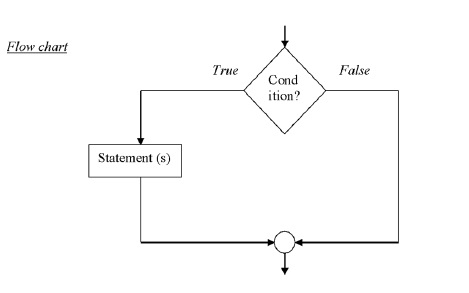## Example

The following example checks whether a number is greater than 50.

#include<stdio.h>
main (){
int a;
printf (“enter any number:”);
scanf (“%d”, &a);
if (a>50)
printf (“%d is greater than 50”, a);
}

## Output

1) enter any number: 60
60 is greater than 50 .
2) enter any number 20
no output

## The if else statement

The if –else statement takes either True or False condition.

### Syntax

if (condition){
True block statement(s)
}
else{
False block statement(s)
}

### Flowchart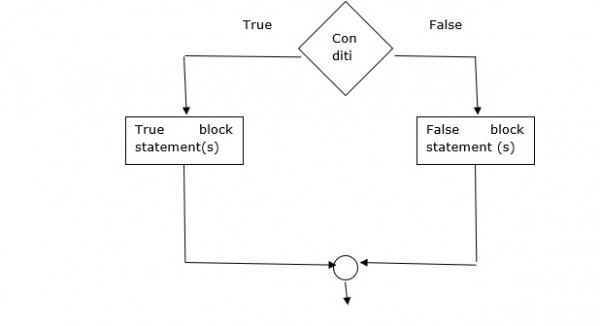## Example

Following is the program to check for even or odd number −

#include<stdio.h>
main (){
int n;
printf (“enter any number:”);
scanf (“%d”, &n);
if (n%2 ==0)
printf (“%d is even number”, n);
else
printf( “%d is odd number”, n);
}

## Output

1) enter any number: 10
10 is even number

## Nested if - else statement

Here ‘if’ is placed inside another if (or) else −

### Syntax

if (condition1){
if (condition2)
stmt1;
else
stmt2;
}
else{
if (condition3)
stmt3;
else
stmt4;
}

### Flowchart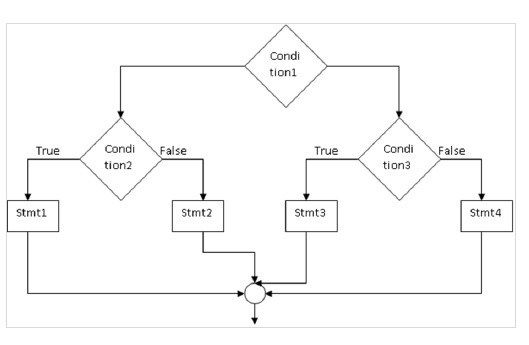## Example

Following example is to print the largest of 3 numbers from the given numbers −

#include<stdio.h>
main (){
int a,b,c;
printf (“enter 3 numbers”);
scanf (“%d%d%d”, &a, &b, &c);
if (a>b){
if (a>c)
printf (“%d is largest”, a);
else
printf (“%d is largest”, c);
} else {
if (b>c)
printf (“%d is largest”, b);
else
printf (“%d is largest”, c);
}
}

## Output

enter 3 numbers = 10 20 30
30 is largest

It is a multi-way decision condition.

### Syntax

if (condition1)
stmt1;
else if (condition2)
stmt2;
- - - - -
- - - - -
else if (condition n)
stmt n;
else
stmt x;

### Flowchart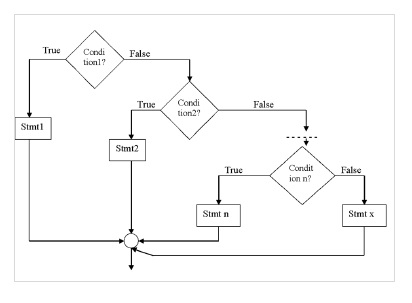## Example

Following example finds roots of quadratic equation −

#include <math.h>
main (){
int a,b,c,d;
float r1, r2
printf ("enter the values a b c");
scanf (“%d%d%d”, &a, &b, &c);
d= b*b – 4*a*c ;
if (d>0){
r1 = (-b+sqrt(d)) / (2*a);
r2 = (-b-sqrt(d)) / (2*a);
printf (“root1 ,root2 =%f%f”, r1, r2);
}
else if (d== 0){
r1 = -b / (2*a);
r2 = -b/ (2*a);
printf (“root1, root2 = %f%f”, r1, r2);
}
else
printf ("roots are imaginary”);
}

## Output

1) enter the values of a b c : 1 4 3
Root 1 = -1
Root 2 = -3

## Switch statement

It is helpful in selecting one among multiple decisions.

### Syntax

switch (expression){
case value1 : stmt1;
break;
case value2 : stmt2;
break;
- - - - - -
default : stmt – x;
}

### Syntax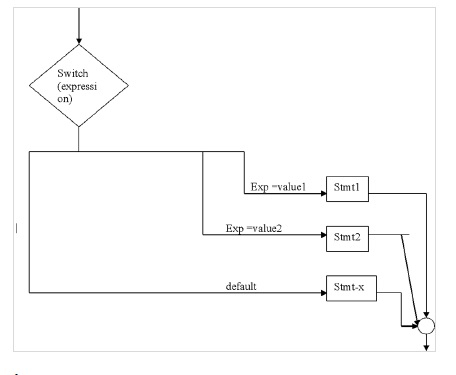## Example

#include<stdio.h>
main (){
int n;
printf (“enter a number”);
scanf (“%d”, &n);
switch (n){
case 0 : printf (“zero”)
break;
case 1 : printf (‘one”);
break;
default : printf (‘wrong choice”);
}
}

## Output

enter a number
1
One